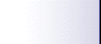How to compute the DFCC Since this list was created we felt the need of a stimulent to make this list more exciting than a picture exposition. Everyone is now rated as it happens in chess tournaments. Each member has a quotha called DFCC (or DTJC, in english: Dexterity in Tricking the Judges' Comitee). The DFCC is mesured in Dexters (Dex for short). You start being rated after the first solution is submitted. Also, you may ask to stop the rating process by sending a mail at probleme-owner@ldc.ro clearly stating your intention. This address may also be used to restart the rating, but please don't use this every time your DFCC goes low. After each deadline, your DFCC will change. This change depends on how well you have done. If you do not submit a solution to a problem, your DFCC will lose 10 dexters. The mathematical laws of DFCC computing follow. When you send the first source, your DFCC is set to 1000. Each problem will have its its own "basic rating" P (a medium-rated problem will have around 50 dex, while a difficult one will may have up to 100 dex). The value of P is contained in the problem's text. The problem, will not be judged equally for all contestants, because its my belief that if a low rated member solves a problem, he should be rated better than a more experienced collegue. The maximum amount of Dexters that can be gained by a contestant with the DFCC equal to D by solving a problem rated P is: DMax = min (1000*P/D, 3*P) In other words, a new-commer (having D=1000) may earn 1000*P/1000 = P dexteri, while one with a lower DFCC (for example D=500) may receive for the same problem 1000*P/500=2*P dexters. As you can see from the formula above, one cannot get more that 3*P. Of course, the DFCC may also decrease. The maximum amount of Dexters one can lose (if he doesn't pass any tests) is: DMin = (D*P/3000) So, if you have your DFCC equal to 1000, you would lose 33% of P, while if your DFCC is 1500 you would lose 50% of P. "The bigger they are, the harder they fall" :) We must also consider the number of tests for every problem. If a contestant passes NTE tests, his DFCC would remain constant. If he passes more that NTE tests, it gains Dexters; if he passes less, it loses Dexters. The NTE grows with the DFCC, so a good programmer may have to pass 75% of tests, while for a medium one 30% will do. Here is how we compute the NTE: NTE = min( [3*D*T/10000], [90%*T]) ([x] = the integer part of x) where T is the number of tests. To give an example, let's try to see what happens for the usual T (T=10). If you are a biginner (D=1000) you must pass NTE=[3*1000*10/10000]=3 tests to mentain your, DFCC. If you are better skilled (D=1700) you must passNTE=[3*1700*10/10000]=5 teste. The number of tests to be passed was limited to 90% so the genius programmer won't have to pass all test to mentain their position. Now let's see what happens between 0 and NTE, and between NTE and T. Naturally we make a linear distribution. E.g.: let's say that we get DMin=50 Dexters, DMax=72 Dexters and NTE=4 tests (out of the total of 10). Then we have: ``` Passed tests: 0 1 2 3 4 5 6 7 8 9 10 Dexters received:-50 -37 -25 -12 0 12 24 36 48 60 72 ``` To make everything clear, here is a table showing the values of Dmax and Dmin for different values of DFCC, for a problem rated with P=100 Dexters and T=10 tests (Dmax and Dmin are real numbers. They are rounded after all the calculus is done): ``` DFCC NTE DMax DMin 0 0 300 0 500 1 200 16 1000 3 100 33 1500 4 66 50 2000 6 50 66 2500 7 40 83 3000 9 33 100 3500 9 28 116 4000 9 25 133 ``` In other words, for P=100 Dexters, and DFCC=2500 Dexters one may gain at most 40 Dexters, but also may lose up to 83 Dexters (if fails all tests). It T=10, one is required to pass 7 tests.